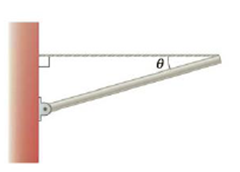Chapter 8, Problem 30P

Chapter
Section
Textbook Problem

A strut of length L = 3.00 m and mass m = 16.0 kg is held by a cable at an angle of θ = 30.0° with respect to the horizontal as shown in Figure P8.30. (a) Sketch a force diagram, indicating all the forces and their placement on the strut, (b) Why is the hinge a good place to use for calculating torques? (c) Write the condition for rotational equilibrium symbolically, calculating the torques around the hinge, (d) Use the torque equation to calculate the tension in the cable, (e) Write the x- and y-components of Newton’s second law for equilibrium. (f) Use the force equation to find the x- and y-components of the force on the hinge, (g) Assuming the strut position is to remain the same, would it be advantageous to attach the cable higher up on the wall? Explain the benefit in terms of the force on the hinge and cable tension.Figure P8.30

(a)

To determine
Draw a force diagram and their placement on the strut.

Explanation

The weight of the beam acting downward from its midpoint, tension of the acting left towards the wall and the horizontal force of the wall on the strut acts towards right and the vertical force of the wall on the strut acts upward.

The Free body diagram for force acting on the strut is given below...

(b)

To determine
Whether the hinge is a good place to use for calculating torques.

(c)

To determine
The condition for rotational equilibrium to calculate torque around the hinge.

(d)

To determine
Tension in the cable byUsing torque equation.

(e)

To determine
The x- and y-components of Newton's second law for equilibrium.

(f)

To determine
The x- and y-components of the force on the hinge.

(g)

To determine
The advantages, if the cable is attached higher up on the wall.

Still sussing out bartleby?

Check out a sample textbook solution.

See a sample solution

The Solution to Your Study Problems

Bartleby provides explanations to thousands of textbook problems written by our experts, many with advanced degrees!

Get Started